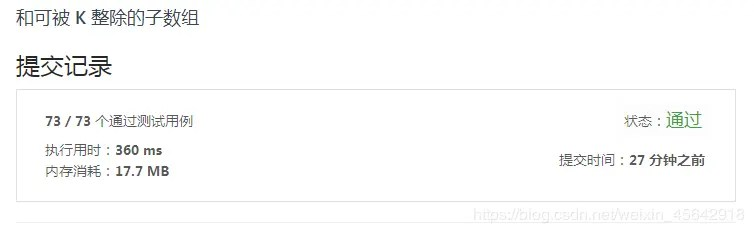# LeetCode 974. and sub-arrays divisible by K | Python

Posted May 27, 20203 min read

### 974 . And a subarray divisible by K

Source of the question:LeetCode [ https://leetcode-cn.com/problems/subarray-sums-divisible-by-k] ( https://leetcode-cn.com/problems/subarray-sums-divisible- by-k)

#### Title

Given an integer array A, return the number of(continuous, non-empty) sub-arrays where the sum of the elements is divisible by K.

Example:

``````Input:A = [4,5,0, -2, -3,1], K = 5
Output:7
Explanation:
There are 7 sub-arrays that satisfy the sum of its elements divisible by K = 5:
[4, 5, 0, -2, -3, 1], , [5, 0], [5, 0, -2, -3], , [0, -2, -3], [-twenty three]``````

prompt:

• 1 <= A.length <= 30000
• \ -10000 <= A \ [i ]<= 10000
• 2 <= K <= 10000

#### Problem solving ideas

Thinking:prefix and

First of all, let me explain in advance. Regarding division and rounding, Python uses rounding down. There may be deviations from expectations, for example, when the divisor is negative. See the following example:

``````>>> 10 //3
3
>>> 10%3
1
>>> 10 //-3
-4
>>> 10%-3
-2``````

It can be seen here that regarding the rounding of positive numbers, the modulus will not deviate too much from the expectation. But for negative numbers, the result may not be as expected. This is because, as mentioned earlier, Python uses rounding down.

For 10 ÷ -3 = -3.3333, then round down to get -4, then the remainder is -2. This is why the above results appear. This section only gives some tips.

In this question, K, here is the divisor, and the title explains [2 <= K <= 10000], so there will not be the situation described above. But the result of taking the remainder of negative numbers in Python is a positive number, which is different from some other programming languages. In some languages, the result of taking the remainder of a negative number is a negative number, so additional processing is required.

This section uses the idea of prefix sum. Regarding the prefix sum, a general explanation.

If the sum of the first i items is required, then:

`P [i]= A + A + ... + A [i]`

The corresponding sum of the first i-1 items is:

`P [i-1]= A + A + ... + A [i-1]`

Then, the value of A \ [i ]can also be expressed as

`A [i]= P [i]-P [i-1]`

Correspondingly, if you want to calculate the sum of consecutive sub-arrays from item i to item j, you can also write the following form:

`sum [i ... j]= P [j]-P [i-1], where(0 <i <j)`

Then the requirement in the question is to determine whether the sum of the sub-arrays is divisible by K, which is now equivalent to determining whether(P \ [j ]-P \ [i-1 ]) mod K == 0 holds.

Here, an additional theorem is mentioned:congruence theorem.

Congruence Theorem:Given a positive integer m, if two integers a and b satisfy a-b can be divisible by m, that is(a-b)/m to get an integer, then it is said that integer a and b are modulo m congruent.

Then the above formula that needs to be judged can also be converted to find whether P \ [j ]mod K == P \ [i-1 ]mod K formula is true.

Specific methods:

• Maintain a hash table, where hash table key is the value of prefix and modulo K, and value is the number of occurrences.

• Traverse each item of the array, find the current prefix and modulo K, and store it in the hash table

• When it does not exist in the table, save the key and value
• When present, the value of the corresponding key +1
• While traversing, make statistics. If the key in the hash table is equal to the value of the current prefix and modulo k:

• Explain that the value of the prefix and modulo K are the same as the value calculated this time
• Accumulate the number of times the key that meets the condition appears in the result

The specific code implementation is as follows.

#### Code

``````class Solution:
def subarraysDivByK(self, A:List [int], K:int)-> int:
# Here is the case where the prefix and itself are divisible by K
hashmap = {0:1}
pre_sum = 0
cnt = 0

for i in range(len(A)):
pre_sum + = A [i]
# Modulo
mod = pre_sum%K
# Use dictionary get method here
# When there is the same key, add to cnt
if mod in hashmap:
cnt + = hashmap [mod]
hashmap [mod]+ = 1
# If the key is in the hash table, add 1 to the number of times,
# Otherwise initialize to 1
else:
hashmap [mod]= 1

return cnt``````

#### ResultsThe above is the idea of using prefix sum to solve the main content of the problem of "974. and sub-arrays divisible by K".

Welcome to the WeChat public account "Book Collection"